Skip to content
{{ message }}

Instantly share code, notes, and snippets.

# igv/KrigBilateral.glsl

Last active Apr 25, 2021
Good test pattern: https://www.rtings.com/images/test-materials/2017/chroma-444.png (Compress it with any lossy codec first, for example jpeg. You can do it with mpv, only add screenshot-jpeg-source-chroma=no to mpv.conf). Usage: glsl-shader="~~/KrigBilateral.glsl"
 // KrigBilateral by Shiandow // // This library is free software; you can redistribute it and/or // modify it under the terms of the GNU Lesser General Public // License as published by the Free Software Foundation; either // version 3.0 of the License, or (at your option) any later version. // // This library is distributed in the hope that it will be useful, // but WITHOUT ANY WARRANTY; without even the implied warranty of // MERCHANTABILITY or FITNESS FOR A PARTICULAR PURPOSE. See the GNU // Lesser General Public License for more details. // // You should have received a copy of the GNU Lesser General Public // License along with this library. //!HOOK CHROMA //!BIND HOOKED //!BIND LUMA //!SAVE LOWRES_Y //!WIDTH LUMA.w //!WHEN CHROMA.w LUMA.w < //!DESC KrigBilateral Downscaling Y pass 1 #define offset vec2(0) #define axis 1 #define Kernel(x) dot(vec3(0.42659, -0.49656, 0.076849), cos(vec3(0, 1, 2) * acos(-1.) * (x + 1.))) vec4 hook() { // Calculate bounds float low = ceil((LUMA_pos - CHROMA_pt) * LUMA_size - offset - 0.5)[axis]; float high = floor((LUMA_pos + CHROMA_pt) * LUMA_size - offset - 0.5)[axis]; float W = 0.0; vec4 avg = vec4(0); vec2 pos = LUMA_pos; for (float k = low; k <= high; k++) { pos[axis] = LUMA_pt[axis] * (k - offset[axis] + 0.5); float rel = (pos[axis] - LUMA_pos[axis])*CHROMA_size[axis]; float w = Kernel(rel); vec4 y = textureGrad(LUMA_raw, pos, vec2(0.0), vec2(0.0)).xxxx * LUMA_mul; y.y *= y.y; avg += w * y; W += w; } avg /= W; avg.y = abs(avg.y - avg.x * avg.x); return avg; } //!HOOK CHROMA //!BIND HOOKED //!BIND LOWRES_Y //!SAVE LOWRES_Y //!WHEN CHROMA.w LUMA.w < //!DESC KrigBilateral Downscaling Y pass 2 #define offset vec2(0) #define axis 0 #define Kernel(x) dot(vec3(0.42659, -0.49656, 0.076849), cos(vec3(0, 1, 2) * acos(-1.) * (x + 1.))) vec4 hook() { // Calculate bounds float low = ceil((LOWRES_Y_pos - CHROMA_pt) * LOWRES_Y_size - offset - 0.5)[axis]; float high = floor((LOWRES_Y_pos + CHROMA_pt) * LOWRES_Y_size - offset - 0.5)[axis]; float W = 0.0; vec4 avg = vec4(0); vec2 pos = LOWRES_Y_pos; for (float k = low; k <= high; k++) { pos[axis] = LOWRES_Y_pt[axis] * (k - offset[axis] + 0.5); float rel = (pos[axis] - LOWRES_Y_pos[axis])*CHROMA_size[axis]; float w = Kernel(rel); vec4 y = textureGrad(LOWRES_Y_raw, pos, vec2(0.0), vec2(0.0)).xxxx * LOWRES_Y_mul; y.y *= y.y; avg += w * y; W += w; } avg /= W; avg.y = abs(avg.y - avg.x * avg.x) + LOWRES_Y_texOff(0).y; return avg; } //!HOOK CHROMA //!BIND HOOKED //!BIND LUMA //!BIND LOWRES_Y //!WIDTH LUMA.w //!HEIGHT LUMA.h //!WHEN CHROMA.w LUMA.w < //!OFFSET ALIGN //!DESC KrigBilateral Upscaling UV #define sqr(x) dot(x,x) #define sigma_nsq 256.0/(255.0*255.0) #define N 8 #define M(i,j) Mx[min(i,j)*N + max(i,j) - (min(i,j)*(min(i,j)+1))/2] #define C(i,j) (inversesqrt(1.0 + (X[i].y + X[j].y)/localVar) * exp(-0.5*(sqr(X[i].x - X[j].x)/(localVar + X[i].y + X[j].y) + sqr((coords[i] - coords[j])/radius))) + (X[i].x - y) * (X[j].x - y) / localVar) #define c(i) (inversesqrt(1.0 + X[i].y/localVar) * exp(-0.5*(sqr(X[i].x - y)/(localVar + X[i].y) + sqr((coords[i] - offset)/radius)))) #define getnsum(i) X[i] = vec4(LOWRES_Y_tex(LOWRES_Y_pt*(pos+coords[i]+vec2(0.5))).xy, \ CHROMA_tex(CHROMA_pt*(pos+coords[i]+vec2(0.5))).xy); \ w = clamp(1.5 - abs(coords[i] - offset), 0.0, 1.0); \ total += w.x*w.y*vec4(X[i].x, X[i].x * X[i].x, X[i].y, 1.0); #define I3(f, n) f(n) f(n+1) f(n+2) #define I9(f, n) I3(f, n) I3(f, n+3) I3(f, n+6) vec4 hook() { vec2 pos = CHROMA_pos * HOOKED_size - vec2(0.5); vec2 offset = pos - round(pos); pos -= offset; vec2 coords[N+1]; vec4 X[N+1]; vec2 w; vec4 total = vec4(0); coords = vec2(-1,-1); coords = vec2(-1, 0); coords = vec2(-1, 1); coords = vec2( 0,-1); coords = vec2( 0, 1); coords = vec2( 1,-1); coords = vec2( 1, 0); coords = vec2( 1, 1); coords = vec2( 0, 0); I9(getnsum, 0) total.xyz /= total.w; float localVar = sigma_nsq + abs(total.y - total.x * total.x) + total.z; float radius = 1.0; float y = LUMA_texOff(0).x; float Mx[(N*(N+1))/2]; float b[N]; vec2 interp = X[N].zw; b = c(0) - c(N) - C(0,N) + C(N,N); M(0, 0) = C(0,0) - C(0,N) - C(0,N) + C(N,N); M(0, 1) = C(0,1) - C(1,N) - C(0,N) + C(N,N); M(0, 2) = C(0,2) - C(2,N) - C(0,N) + C(N,N); M(0, 3) = C(0,3) - C(3,N) - C(0,N) + C(N,N); M(0, 4) = C(0,4) - C(4,N) - C(0,N) + C(N,N); M(0, 5) = C(0,5) - C(5,N) - C(0,N) + C(N,N); M(0, 6) = C(0,6) - C(6,N) - C(0,N) + C(N,N); M(0, 7) = C(0,7) - C(7,N) - C(0,N) + C(N,N); b = c(1) - c(N) - C(1,N) + C(N,N); M(1, 1) = C(1,1) - C(1,N) - C(1,N) + C(N,N); M(1, 2) = C(1,2) - C(2,N) - C(1,N) + C(N,N); M(1, 3) = C(1,3) - C(3,N) - C(1,N) + C(N,N); M(1, 4) = C(1,4) - C(4,N) - C(1,N) + C(N,N); M(1, 5) = C(1,5) - C(5,N) - C(1,N) + C(N,N); M(1, 6) = C(1,6) - C(6,N) - C(1,N) + C(N,N); M(1, 7) = C(1,7) - C(7,N) - C(1,N) + C(N,N); b = c(2) - c(N) - C(2,N) + C(N,N); M(2, 2) = C(2,2) - C(2,N) - C(2,N) + C(N,N); M(2, 3) = C(2,3) - C(3,N) - C(2,N) + C(N,N); M(2, 4) = C(2,4) - C(4,N) - C(2,N) + C(N,N); M(2, 5) = C(2,5) - C(5,N) - C(2,N) + C(N,N); M(2, 6) = C(2,6) - C(6,N) - C(2,N) + C(N,N); M(2, 7) = C(2,7) - C(7,N) - C(2,N) + C(N,N); b = c(3) - c(N) - C(3,N) + C(N,N); M(3, 3) = C(3,3) - C(3,N) - C(3,N) + C(N,N); M(3, 4) = C(3,4) - C(4,N) - C(3,N) + C(N,N); M(3, 5) = C(3,5) - C(5,N) - C(3,N) + C(N,N); M(3, 6) = C(3,6) - C(6,N) - C(3,N) + C(N,N); M(3, 7) = C(3,7) - C(7,N) - C(3,N) + C(N,N); b = c(4) - c(N) - C(4,N) + C(N,N); M(4, 4) = C(4,4) - C(4,N) - C(4,N) + C(N,N); M(4, 5) = C(4,5) - C(5,N) - C(4,N) + C(N,N); M(4, 6) = C(4,6) - C(6,N) - C(4,N) + C(N,N); M(4, 7) = C(4,7) - C(7,N) - C(4,N) + C(N,N); b = c(5) - c(N) - C(5,N) + C(N,N); M(5, 5) = C(5,5) - C(5,N) - C(5,N) + C(N,N); M(5, 6) = C(5,6) - C(6,N) - C(5,N) + C(N,N); M(5, 7) = C(5,7) - C(7,N) - C(5,N) + C(N,N); b = c(6) - c(N) - C(6,N) + C(N,N); M(6, 6) = C(6,6) - C(6,N) - C(6,N) + C(N,N); M(6, 7) = C(6,7) - C(7,N) - C(6,N) + C(N,N); b = c(7) - c(N) - C(7,N) + C(N,N); M(7, 7) = C(7,7) - C(7,N) - C(7,N) + C(N,N); b -= b * M(1, 0) / M(0, 0); M(1, 1) -= M(0, 1) * M(1, 0) / M(0, 0); M(1, 2) -= M(0, 2) * M(1, 0) / M(0, 0); M(1, 3) -= M(0, 3) * M(1, 0) / M(0, 0); M(1, 4) -= M(0, 4) * M(1, 0) / M(0, 0); M(1, 5) -= M(0, 5) * M(1, 0) / M(0, 0); M(1, 6) -= M(0, 6) * M(1, 0) / M(0, 0); M(1, 7) -= M(0, 7) * M(1, 0) / M(0, 0); b -= b * M(2, 0) / M(0, 0); M(2, 2) -= M(0, 2) * M(2, 0) / M(0, 0); M(2, 3) -= M(0, 3) * M(2, 0) / M(0, 0); M(2, 4) -= M(0, 4) * M(2, 0) / M(0, 0); M(2, 5) -= M(0, 5) * M(2, 0) / M(0, 0); M(2, 6) -= M(0, 6) * M(2, 0) / M(0, 0); M(2, 7) -= M(0, 7) * M(2, 0) / M(0, 0); b -= b * M(3, 0) / M(0, 0); M(3, 3) -= M(0, 3) * M(3, 0) / M(0, 0); M(3, 4) -= M(0, 4) * M(3, 0) / M(0, 0); M(3, 5) -= M(0, 5) * M(3, 0) / M(0, 0); M(3, 6) -= M(0, 6) * M(3, 0) / M(0, 0); M(3, 7) -= M(0, 7) * M(3, 0) / M(0, 0); b -= b * M(4, 0) / M(0, 0); M(4, 4) -= M(0, 4) * M(4, 0) / M(0, 0); M(4, 5) -= M(0, 5) * M(4, 0) / M(0, 0); M(4, 6) -= M(0, 6) * M(4, 0) / M(0, 0); M(4, 7) -= M(0, 7) * M(4, 0) / M(0, 0); b -= b * M(5, 0) / M(0, 0); M(5, 5) -= M(0, 5) * M(5, 0) / M(0, 0); M(5, 6) -= M(0, 6) * M(5, 0) / M(0, 0); M(5, 7) -= M(0, 7) * M(5, 0) / M(0, 0); b -= b * M(6, 0) / M(0, 0); M(6, 6) -= M(0, 6) * M(6, 0) / M(0, 0); M(6, 7) -= M(0, 7) * M(6, 0) / M(0, 0); b -= b * M(7, 0) / M(0, 0); M(7, 7) -= M(0, 7) * M(7, 0) / M(0, 0); b -= b * M(2, 1) / M(1, 1); M(2, 2) -= M(1, 2) * M(2, 1) / M(1, 1); M(2, 3) -= M(1, 3) * M(2, 1) / M(1, 1); M(2, 4) -= M(1, 4) * M(2, 1) / M(1, 1); M(2, 5) -= M(1, 5) * M(2, 1) / M(1, 1); M(2, 6) -= M(1, 6) * M(2, 1) / M(1, 1); M(2, 7) -= M(1, 7) * M(2, 1) / M(1, 1); b -= b * M(3, 1) / M(1, 1); M(3, 3) -= M(1, 3) * M(3, 1) / M(1, 1); M(3, 4) -= M(1, 4) * M(3, 1) / M(1, 1); M(3, 5) -= M(1, 5) * M(3, 1) / M(1, 1); M(3, 6) -= M(1, 6) * M(3, 1) / M(1, 1); M(3, 7) -= M(1, 7) * M(3, 1) / M(1, 1); b -= b * M(4, 1) / M(1, 1); M(4, 4) -= M(1, 4) * M(4, 1) / M(1, 1); M(4, 5) -= M(1, 5) * M(4, 1) / M(1, 1); M(4, 6) -= M(1, 6) * M(4, 1) / M(1, 1); M(4, 7) -= M(1, 7) * M(4, 1) / M(1, 1); b -= b * M(5, 1) / M(1, 1); M(5, 5) -= M(1, 5) * M(5, 1) / M(1, 1); M(5, 6) -= M(1, 6) * M(5, 1) / M(1, 1); M(5, 7) -= M(1, 7) * M(5, 1) / M(1, 1); b -= b * M(6, 1) / M(1, 1); M(6, 6) -= M(1, 6) * M(6, 1) / M(1, 1); M(6, 7) -= M(1, 7) * M(6, 1) / M(1, 1); b -= b * M(7, 1) / M(1, 1); M(7, 7) -= M(1, 7) * M(7, 1) / M(1, 1); b -= b * M(3, 2) / M(2, 2); M(3, 3) -= M(2, 3) * M(3, 2) / M(2, 2); M(3, 4) -= M(2, 4) * M(3, 2) / M(2, 2); M(3, 5) -= M(2, 5) * M(3, 2) / M(2, 2); M(3, 6) -= M(2, 6) * M(3, 2) / M(2, 2); M(3, 7) -= M(2, 7) * M(3, 2) / M(2, 2); b -= b * M(4, 2) / M(2, 2); M(4, 4) -= M(2, 4) * M(4, 2) / M(2, 2); M(4, 5) -= M(2, 5) * M(4, 2) / M(2, 2); M(4, 6) -= M(2, 6) * M(4, 2) / M(2, 2); M(4, 7) -= M(2, 7) * M(4, 2) / M(2, 2); b -= b * M(5, 2) / M(2, 2); M(5, 5) -= M(2, 5) * M(5, 2) / M(2, 2); M(5, 6) -= M(2, 6) * M(5, 2) / M(2, 2); M(5, 7) -= M(2, 7) * M(5, 2) / M(2, 2); b -= b * M(6, 2) / M(2, 2); M(6, 6) -= M(2, 6) * M(6, 2) / M(2, 2); M(6, 7) -= M(2, 7) * M(6, 2) / M(2, 2); b -= b * M(7, 2) / M(2, 2); M(7, 7) -= M(2, 7) * M(7, 2) / M(2, 2); b -= b * M(4, 3) / M(3, 3); M(4, 4) -= M(3, 4) * M(4, 3) / M(3, 3); M(4, 5) -= M(3, 5) * M(4, 3) / M(3, 3); M(4, 6) -= M(3, 6) * M(4, 3) / M(3, 3); M(4, 7) -= M(3, 7) * M(4, 3) / M(3, 3); b -= b * M(5, 3) / M(3, 3); M(5, 5) -= M(3, 5) * M(5, 3) / M(3, 3); M(5, 6) -= M(3, 6) * M(5, 3) / M(3, 3); M(5, 7) -= M(3, 7) * M(5, 3) / M(3, 3); b -= b * M(6, 3) / M(3, 3); M(6, 6) -= M(3, 6) * M(6, 3) / M(3, 3); M(6, 7) -= M(3, 7) * M(6, 3) / M(3, 3); b -= b * M(7, 3) / M(3, 3); M(7, 7) -= M(3, 7) * M(7, 3) / M(3, 3); b -= b * M(5, 4) / M(4, 4); M(5, 5) -= M(4, 5) * M(5, 4) / M(4, 4); M(5, 6) -= M(4, 6) * M(5, 4) / M(4, 4); M(5, 7) -= M(4, 7) * M(5, 4) / M(4, 4); b -= b * M(6, 4) / M(4, 4); M(6, 6) -= M(4, 6) * M(6, 4) / M(4, 4); M(6, 7) -= M(4, 7) * M(6, 4) / M(4, 4); b -= b * M(7, 4) / M(4, 4); M(7, 7) -= M(4, 7) * M(7, 4) / M(4, 4); b -= b * M(6, 5) / M(5, 5); M(6, 6) -= M(5, 6) * M(6, 5) / M(5, 5); M(6, 7) -= M(5, 7) * M(6, 5) / M(5, 5); b -= b * M(7, 5) / M(5, 5); M(7, 7) -= M(5, 7) * M(7, 5) / M(5, 5); b -= b * M(7, 6) / M(6, 6); M(7, 7) -= M(6, 7) * M(7, 6) / M(6, 6); b /= M(7, 7); interp += b * (X - X[N]).zw; b -= M(6, 7) * b; b /= M(6, 6); interp += b * (X - X[N]).zw; b -= M(5, 6) * b; b -= M(5, 7) * b; b /= M(5, 5); interp += b * (X - X[N]).zw; b -= M(4, 5) * b; b -= M(4, 6) * b; b -= M(4, 7) * b; b /= M(4, 4); interp += b * (X - X[N]).zw; b -= M(3, 4) * b; b -= M(3, 5) * b; b -= M(3, 6) * b; b -= M(3, 7) * b; b /= M(3, 3); interp += b * (X - X[N]).zw; b -= M(2, 3) * b; b -= M(2, 4) * b; b -= M(2, 5) * b; b -= M(2, 6) * b; b -= M(2, 7) * b; b /= M(2, 2); interp += b * (X - X[N]).zw; b -= M(1, 2) * b; b -= M(1, 3) * b; b -= M(1, 4) * b; b -= M(1, 5) * b; b -= M(1, 6) * b; b -= M(1, 7) * b; b /= M(1, 1); interp += b * (X - X[N]).zw; b -= M(0, 1) * b; b -= M(0, 2) * b; b -= M(0, 3) * b; b -= M(0, 4) * b; b -= M(0, 5) * b; b -= M(0, 6) * b; b -= M(0, 7) * b; b /= M(0, 0); interp += b * (X - X[N]).zw; return interp.xyxy; }

###CrisBRM commented Sep 8, 2016 • edited

 Last review broke it and crashes the driver (radeon, 280x). Jun 21 crossbilateral is the last one to work flawlessly. Edit: updating mesa and llvm to -git has finally fixed it, BUT the performance decrease is gargantuan.

###CrisBRM commented Oct 22, 2016

 Does setting cscale to anything other than bilinear matter?

###igv commented Oct 23, 2016

 No need to set cscale to anything.

###CrisBRM commented Apr 21, 2017

 Latest review changed render times from 3,500 to 30,000 while playing a 1080p video.

###roberth1990 commented Aug 10, 2017

 How does it compare to ewa_lanczossharp?

###igv commented Sep 3, 2017 • edited

 ewa_lanczos and ewa_lanczossharp are almost the same thing.

###igv commented Nov 5, 2018 • edited

 It's now better quality and much faster (especially under vulkan) after the last update.

###fingerpad commented Mar 1, 2019

 krig causes artifacts with software decoded 10 bit HEVC and AVC video if debanding is not enabled. /g/ thread with screenshots and information: https://rbt.asia/g/thread/69957207/#69993472

###igv commented Mar 2, 2019

 Should be fixed now.

###fingerpad commented Mar 3, 2019

 thank you!

###deus0ww commented Mar 22, 2019

 What's a reasonable range for locality? How high should it be set for maximum sharpness?

###igv commented Mar 23, 2019

 How high should it be set for maximum sharpness? 100

###xXedgelordXx commented Mar 28, 2019

 Should I set cscale to bilinear when using krig?

###igv commented Mar 28, 2019

 You can, but not necessary.

###Tsubajashi commented May 7, 2019 • edited

 Do the changes mean, if i want to use it with SSimSuperRes, that i need to set the multiply to 2.0?

###igv commented May 7, 2019

 SSSR is not a luma prescaler. FSRCNN/RAVU/nnedi3 are luma prescalers. Changing offset is not that necessary if are using it with a 2x prescaler though - quality will be only slightly worse.

###Tsubajashi commented May 7, 2019

 that sounds good. i wanted to have a ravu setup sometime. what would be the best thing to use if i want to use ravu-lite with krigbilateral?

###igv commented May 7, 2019 • edited

 Create a copy of the krigbilateral shader. In the 2nd copy (for example called KrigBilateral1.glsl) set OFFSET to -1 0 and replace all `//!WHEN CHROMA.w LUMA.w <` with `//!WHEN CHROMA.w 2.8 * LUMA.w <`. Then use it like this: ``````glsl-shader="~~/ravu-lite.hook" glsl-shader="~~/KrigBilateral1.glsl" glsl-shader="~~/KrigBilateral.glsl" `````` UPDATE: don't need to do this anymore

###crazysword1 commented May 18, 2019 • edited

 "When used with a luma prescaler, multiply the first OFFSET value (the one that is -0.5 by default) by a prescaling factor (due to mpv shader API limitations, offsets can't be calculated automatically)." Sorry I have a little trouble following this and would like your help. Currently using FSRCNNX + KrigBilateral +SSIM + adaptive sharpen So what do I need to edit in the KrigBilateral? Also in my setup, would SSSR be needed? For example if I running at 3840 * 2160 and my video is 720 * 480 the FSRCNNX prescale would double the resolution 1440*960 then would SSSR be utilized to upscale it again? Thanks

###deus0ww commented May 18, 2019

 With FSRCNNX, the offset in line 102 should be changed to "//!OFFSET -1.0 0". What is SSIM? Do you mean SSSR? For 720p -> 4K, you need to scale by 3x. FSRCNNX will do 2x. SSSR can be used for the other 1.5x.

###crazysword1 commented May 18, 2019

 ** With FSRCNNX, the offset in line 102 should be changed to "//!OFFSET -1.0 0". What is SSIM? Do you mean SSSR? For 720p -> 4K, you need to scale by 3x. FSRCNNX will do 2x. SSSR can be used for the other 1.5x. ** Thanks I will change that SSimDownscaler.glsl also made by igv. It's for downscaling. For example if your resolution after 2x upscaling exceeds your monitor resolution, , you will need downscaling. So SSSR will "replace" the mpv built in scaler (e.g. lanczos, spline etc depending on what you have in mpv.conf) ?

###deus0ww commented May 18, 2019

 SSSR enhances the built-in 'scale' setting. SSDS does the same with 'dscale'.

###crazysword1 commented May 19, 2019 • edited

 IGV thanks for your fantastic work in making all these great shaders and sharing it with everyone. Really appreciate what you are doing. I have a suggestion and was wondering what you think. Since FSRCNN was released, new algorithms have came out with better visual quality and slightly lower process time. Notably, MS-LapSRN-D5R2 seems to have significantly better quality with speed that is just lower than FSRCNN. Would you consider writing a new shader for this? The real time quality improvements to upscaled video will make it the best in the world. Thanks again for all your hard work and sharing your wondering creations with us.

###igv commented May 19, 2019

 MS-LapSRN is already a part of FSRCNN-TensorFlow (see LapSRN.py). And it's not faster, MS-LapSRN-D5R2 for 2x upscaling is basically the same as FSRCNNX_x2_64-0-5-2 (it only doesn't support multi-scale model, because it is hard to implement it in a shader).

###crazysword1 commented May 19, 2019 • edited

 Hi IGV, thanks for your quick reply. :) "MS-LapSRN is already a part of FSRCNN-TensorFlow (see LapSRN.py)." Does this mean the current FSRCNNX is already implementing some features of lapsrn? Apology if this is a stupid question. "And it's not faster, MS-LapSRN-D5R2 for 2x upscaling is basically the same as FSRCNNX_x2_64-0-5-2 (it only doesn't support multi-scale model, because it is hard to implement it in a shader)." Sorry what I meant was that MS-LapSRN-D5R2 was slightly slower (guess I was wrong) not faster than FSRCNN. Thanks for letting me know that it would be similar to FSRCNNX_x2_64-0-5-2. I guess even a 2080ti would have a hard time rendering that in real time?

###igv commented May 20, 2019

 Yes Yes

###fingerpad commented May 28, 2019 • edited

 offset should be 0 0 for yuv444 h264/265, right?

 Yes

###deus0ww commented May 29, 2019

 Setting the offset is getting a bit complicated. Could you look at the lua code below and tell me if it's correct? The number after the filename is the offset. ```local function get_scale() return math.min( props['osd-width'] / props['width'], props['osd-height'] / props['height'] ) end local function is_chroma_subsampled() return props['video-params/pixelformat']:find('444') == nil end local function is_chroma_left() return props['video-params/chroma-location'] == 'mpeg2/4/h264' end local function krigbilateral() local scale = get_scale() if is_chroma_left() and is_chroma_subsampled() then if scale < 1.4 then return 'KrigBilateral-05.glsl' end -- No Luma Scaler if scale < 2.828430 then return 'KrigBilateral-10.glsl' end -- 2x Luma Scaler (FSRCNNX) return 'KrigBilateral-20.glsl' -- 4x Luma Scalers (FSRCNNX + RAVU) else return 'KrigBilateral-00.glsl' end end```

###igv commented May 29, 2019 • edited

 ```local function get_scale() return props['osd-width'] / props['dwidth'] end local function is_chroma_subsampled() return props['video-params/pixelformat']:find('444') == nil end local function is_chroma_left() return props['video-params/chroma-location'] == 'mpeg2/4/h264' end local function krigbilateral() local scale = get_scale() if is_chroma_left() and is_chroma_subsampled() then if scale > 3.4 then return 'KrigBilateral-20.glsl' end -- 4x Luma Scaler -- if scale > 2.8 then return 'KrigBilateral-20.glsl' end -- 2x+2x Luma Scalers (FSRCNNX + RAVU) if scale > 2.4 then return 'KrigBilateral-15.glsl' end -- 3x Luma Scaler if scale > 1.4 then return 'KrigBilateral-10.glsl' end -- 2x Luma Scaler (FSRCNNX) return 'KrigBilateral.glsl' -- No Luma Scaler else return 'KrigBilateral-00.glsl' end end``` Something like this. I wouldn't recommend using several prescalers at the same time.

###deus0ww commented May 29, 2019

 Thank you for looking at the code. I don't want to use multiple prescalers but (FSRCNNX + RAVU_lite + Krig + SSimDownscaler) is subjectively the best for 720p -> 4k. If only there were FSRCNNX 3x... Notes: get_scale() also checks height so that it works with vertical/portrait videos. 2.828430 limit for 4x scaling is from 2 * 1/0.707106 (0.707106 is the value used in RAVU's //!WHEN ) The script did not have 3x because there's no 3x prescaler that works on the Mac (RAVU 3x uses compute). The whole script is here: https://github.com/deus0ww/mpv-conf/blob/master/scripts/Shaders.lua

###crazysword1 commented May 30, 2019

 I would like to report a problem When I rotate a video to say 90 degrees, the video will display a purple fringe across the video I have isolated the problem to this shader by testing 1 shader at a time Any ideas why this is happening? I didn't have any problems with the older versions

###igv commented May 31, 2019 • edited

 I fixed that problem, but this shader is still incompatible with video-rotate, again because of mpv shader API limitations (with 90 degrees rotation it should be OFFSET 0 -0.5, with 180 - OFFSET 0.5 0, with 270 - OFFSET 0 0.5). UPDATE: should be compatible with video-rotate on latest mpv.

###crazysword1 commented Jun 1, 2019

 Thanks for fixing this. I can confirm that the problem is gone. I recall you said if I leave the OFFSET at 0 -0.5, the quality would only be slightly worse right?

###deus0ww commented Jun 1, 2019

 In case anyone is interested, I updated my script to dynamically generate and load shader file with correct offsets with regard to chroma-location, chroma-subsampling, prescalers, and rotation (Tested on Macs; probably works on Linux; fails on Windows).

###crazysword1 commented Jun 13, 2019

 Hi IGV, I use most of your shaders in MPV and I was wondering if the order they are listed in mpv.conf makes a difference to performance and quality? What about the "linear downscaling" and "sigmoid=upscaling" lines? Should they be before or after the shaders section? Here is my current setup. Thank you. profile=gpu-hq deband=no gpu-api=vulkan fbo-format=rgba16hf sigmoid-upscaling=no linear-downscaling=no glsl-shader="C:\mpv\mpv\shaders\FSRCNNX_x2_16-0-4-1.glsl" glsl-shader="C:\mpv\mpv\shaders\KrigBilateral.glsl" glsl-shader="C:\mpv\mpv\shaders\SSimSuperRes.glsl" glsl-shader="C:\mpv\mpv\shaders\SSimDownscaler.glsl" glsl-shader="C:\mpv\mpv\shaders\adaptive-sharpen.glsl" scale=lanczos dscale=mitchell cscale=lanczos

###igv commented Jun 14, 2019

 Doesn't make any difference.

###igv commented Jun 14, 2019 • edited

 If your downscaling factor is small (like <2x) you can use `dscale=lanczos` with `linear-downscaling=no` instead of SSimDownscaler.

###crazysword1 commented Jun 14, 2019

 So I guess that means dscale=lanczos vs SSimDownscaler difference is small as long as the factor is <2?

###ganxiao2008 commented Oct 13, 2019

 Hi IGV, krig causes no video output but blue color when hardware decoding 10 bit HEVC video in Mac OS 10.15 if debanding is not enabled. krig enabled + deband=yes -> ok krig disabled + deband=yes -> ok krig disabled + deband=no -> ok krig enabled + deband=no -> fail mpv playback info `````` (+) Video --vid=1 (hevc 3840x2160 59.940fps) (+) Audio --aid=1 (aac) Using hardware decoding (videotoolbox). AO: [coreaudio] 48000Hz stereo 2ch s16 VO: [libmpv] 3840x2160 videotoolbox[p010] `````` shader error log ``````[libmpv_render] fragment shader source: [libmpv_render] [ 1] #version 410 [libmpv_render] [ 2] #define tex1D texture [libmpv_render] [ 3] #define tex3D texture [libmpv_render] [ 4] #define LUT_POS(x, lut_size) mix(0.5 / (lut_size), 1.0 - 0.5 / (lut_size), (x)) [libmpv_render] [ 5] out vec4 out_color; [libmpv_render] [ 6] in vec2 texcoord0; [libmpv_render] [ 7] in vec2 texcoord1; [libmpv_render] [ 8] uniform float random; [libmpv_render] [ 9] uniform int frame; [libmpv_render] [ 10] uniform vec2 input_size; [libmpv_render] [ 11] uniform vec2 target_size; [libmpv_render] [ 12] uniform vec2 tex_offset; [libmpv_render] [ 13] uniform sampler2DRect texture0; [libmpv_render] [ 14] uniform vec2 texture_size0; [libmpv_render] [ 15] uniform mat2 texture_rot0; [libmpv_render] [ 16] uniform vec2 texture_off0; [libmpv_render] [ 17] uniform vec2 pixel_size0; [libmpv_render] [ 18] uniform sampler2DRect texture1; [libmpv_render] [ 19] uniform vec2 texture_size1; [libmpv_render] [ 20] uniform mat2 texture_rot1; [libmpv_render] [ 21] uniform vec2 texture_off1; [libmpv_render] [ 22] uniform vec2 pixel_size1; [libmpv_render] [ 23] #define HOOKED_raw texture0 [libmpv_render] [ 24] #define HOOKED_pos texcoord0 [libmpv_render] [ 25] #define HOOKED_size texture_size0 [libmpv_render] [ 26] #define HOOKED_rot texture_rot0 [libmpv_render] [ 27] #define HOOKED_off texture_off0 [libmpv_render] [ 28] #define HOOKED_pt pixel_size0 [libmpv_render] [ 29] #define HOOKED_map texmap0 [libmpv_render] [ 30] #define HOOKED_mul 1.003906 [libmpv_render] [ 31] #define HOOKED_tex(pos) (HOOKED_mul * vec4(texture(HOOKED_raw, pos)).rgba) [libmpv_render] [ 32] #define HOOKED_texOff(off) HOOKED_tex(HOOKED_pos + HOOKED_pt * vec2(off)) [libmpv_render] [ 33] #define CHROMA_raw texture0 [libmpv_render] [ 34] #define CHROMA_pos texcoord0 [libmpv_render] [ 35] #define CHROMA_size texture_size0 [libmpv_render] [ 36] #define CHROMA_rot texture_rot0 [libmpv_render] [ 37] #define CHROMA_off texture_off0 [libmpv_render] [ 38] #define CHROMA_pt pixel_size0 [libmpv_render] [ 39] #define CHROMA_map texmap0 [libmpv_render] [ 40] #define CHROMA_mul 1.003906 [libmpv_render] [ 41] #define CHROMA_tex(pos) (CHROMA_mul * vec4(texture(CHROMA_raw, pos)).rgba) [libmpv_render] [ 42] #define CHROMA_texOff(off) CHROMA_tex(CHROMA_pos + CHROMA_pt * vec2(off)) [libmpv_render] [ 43] #define LUMA_raw texture1 [libmpv_render] [ 44] #define LUMA_pos texcoord1 [libmpv_render] [ 45] #define LUMA_size texture_size1 [libmpv_render] [ 46] #define LUMA_rot texture_rot1 [libmpv_render] [ 47] #define LUMA_off texture_off1 [libmpv_render] [ 48] #define LUMA_pt pixel_size1 [libmpv_render] [ 49] #define LUMA_map texmap1 [libmpv_render] [ 50] #define LUMA_mul 1.003906 [libmpv_render] [ 51] #define LUMA_tex(pos) (LUMA_mul * vec4(texture(LUMA_raw, pos)).rgba) [libmpv_render] [ 52] #define LUMA_texOff(off) LUMA_tex(LUMA_pos + LUMA_pt * vec2(off)) [libmpv_render] [ 53] [libmpv_render] [ 54] #define lumaOffset (-vec2(0.0, 0.0)*LUMA_size*CHROMA_pt) [libmpv_render] [ 55] [libmpv_render] [ 56] #define axis 1 [libmpv_render] [ 57] [libmpv_render] [ 58] #define Kernel(x) (1. - abs(x)) [libmpv_render] [ 59] [libmpv_render] [ 60] vec4 hook() { [libmpv_render] [ 61] // Calculate bounds [libmpv_render] [ 62] float low = ceil((LUMA_pos - 0.5*CHROMA_pt) * LUMA_size - lumaOffset - 0.5)[axis]; [libmpv_render] [ 63] float high = floor((LUMA_pos + 0.5*CHROMA_pt) * LUMA_size - lumaOffset - 0.5)[axis]; [libmpv_render] [ 64] [libmpv_render] [ 65] float W = 0.0; [libmpv_render] [ 66] vec4 avg = vec4(0); [libmpv_render] [ 67] vec2 pos = LUMA_pos; [libmpv_render] [ 68] [libmpv_render] [ 69] for (float k = low; k <= high; k++) { [libmpv_render] [ 70] pos[axis] = LUMA_pt[axis] * (k - lumaOffset[axis] + 0.5); [libmpv_render] [ 71] float rel = (pos[axis] - LUMA_pos[axis])*CHROMA_size[axis]; [libmpv_render] [ 72] float w = Kernel(rel); [libmpv_render] [ 73] [libmpv_render] [ 74] vec4 y = textureLod(LUMA_raw, pos, 0.0).xxxx * LUMA_mul; [libmpv_render] [ 75] y.y *= y.y; [libmpv_render] [ 76] avg += w * y; [libmpv_render] [ 77] W += w; [libmpv_render] [ 78] } [libmpv_render] [ 79] avg /= W; [libmpv_render] [ 80] avg.y = abs(avg.y - pow(avg.x, 2.0)); [libmpv_render] [ 81] return avg; [libmpv_render] [ 82] } [libmpv_render] [ 83] [libmpv_render] [ 84] void main() { [libmpv_render] [ 85] vec4 color = vec4(0.0, 0.0, 0.0, 1.0); [libmpv_render] [ 86] color = hook(); [libmpv_render] [ 87] color.b = 0.000000; [libmpv_render] [ 88] color.a = 1.000000; [libmpv_render] [ 89] out_color = color; [libmpv_render] [ 90] } [libmpv_render] fragment shader compile log (status=0): [libmpv_render] ERROR: 0:74: No matching function for call to textureLod(sampler2DRect, vec2, float) [libmpv_render] ERROR: 0:75: Use of undeclared identifier 'y' [libmpv_render] ERROR: 0:75: Use of undeclared identifier 'y' [libmpv_render] ERROR: 0:76: Use of undeclared identifier 'y' [libmpv_render] [libmpv_render] shader link log (status=0): ERROR: One or more attached shaders not successfully compiled [libmpv_render] ``````

###Alexkral commented Dec 11, 2019

 Does this work with 4:2:2 and in that case is the first hook needed?

###igv commented Dec 11, 2019

 With 4:2:2 the 1st hook simply copies LUMA to LOWRES_Y. You can remove it, but then you also need to rename all LOWRES_Y to LUMA in the 2nd hook (except line `//!SAVE LOWRES_Y`).

###Alexkral commented Jan 15, 2020

 Hi igv, I have noticed that some of your FSRCNN models produce slight luminance changes that are barely visible when applied to luma, but can become quite obvious in other situations. This affects up to four of the models, the most affected is FSRCNN_x2_r2_8-0-2.glsl. Issues are disabled in the repository, if you contact me I could give you more details that I don't consider appropriate to discuss here.

###igv commented Jan 16, 2020

 FSRCNNX is latest and only supported version. Old models produce slight luminance changes because they were trained with SSIM loss.

###arennf commented May 2, 2020 • edited

 hey igv, do you mind if you reupload the image comparison link at the top? it's not working anymore...

###VEWION commented Dec 22, 2020

 l am having a bad result compare to madvr's Bilateral sharp when applied to these stroke-like image which also common in anime. madvr Bilateral sharp+spline 3 taps: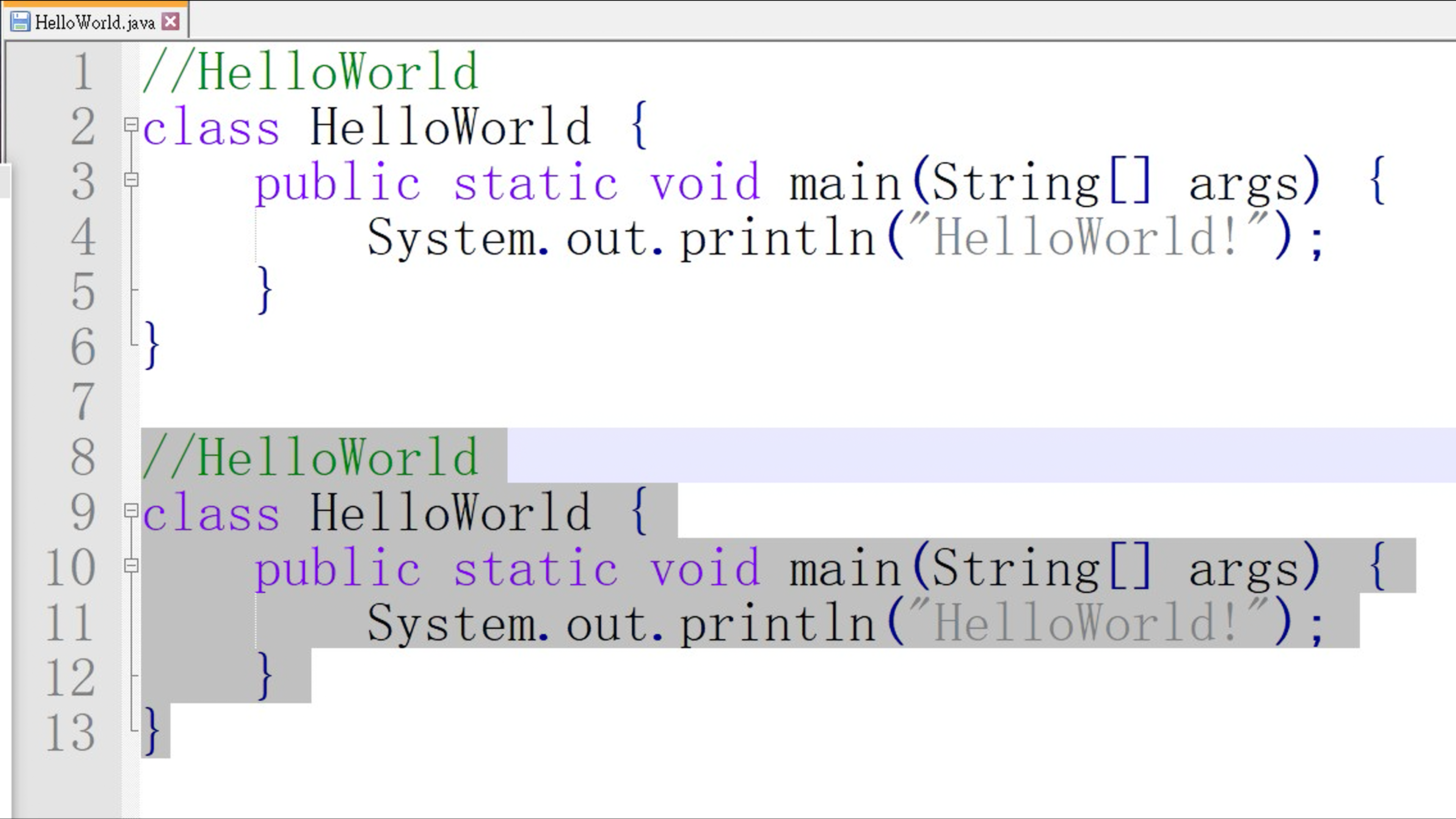mpv KrigBilateral+spline36(profile=gpu-hq):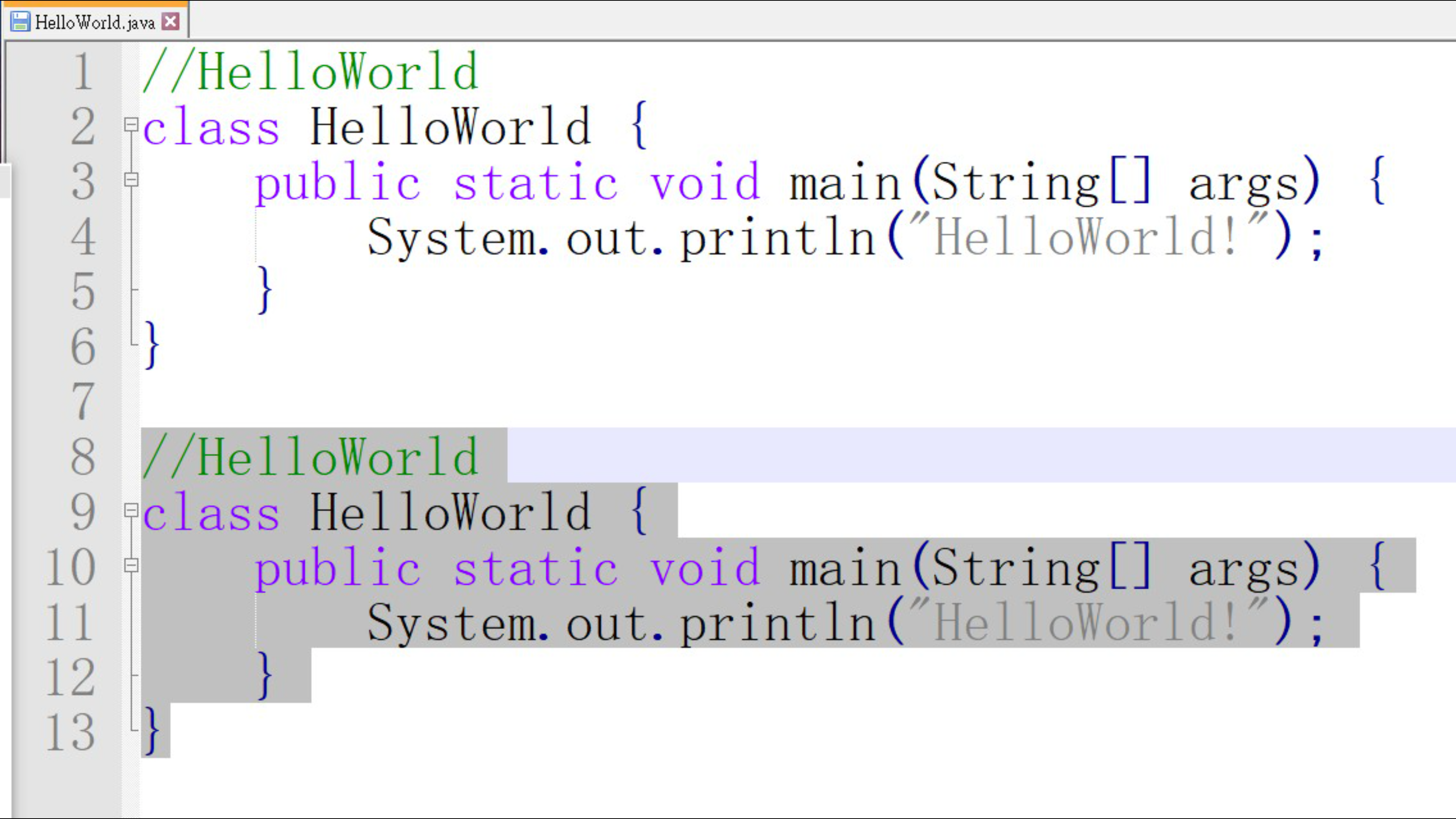madvr(left) mpv(right), I also saw this "red block inside of the stroke" issue in some anime scenes before: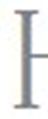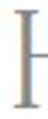###igv commented Dec 22, 2020

 Doesn't look much different than spline36 from mpv (and without jpeg), just sharper. cscale=spline36 + SSSR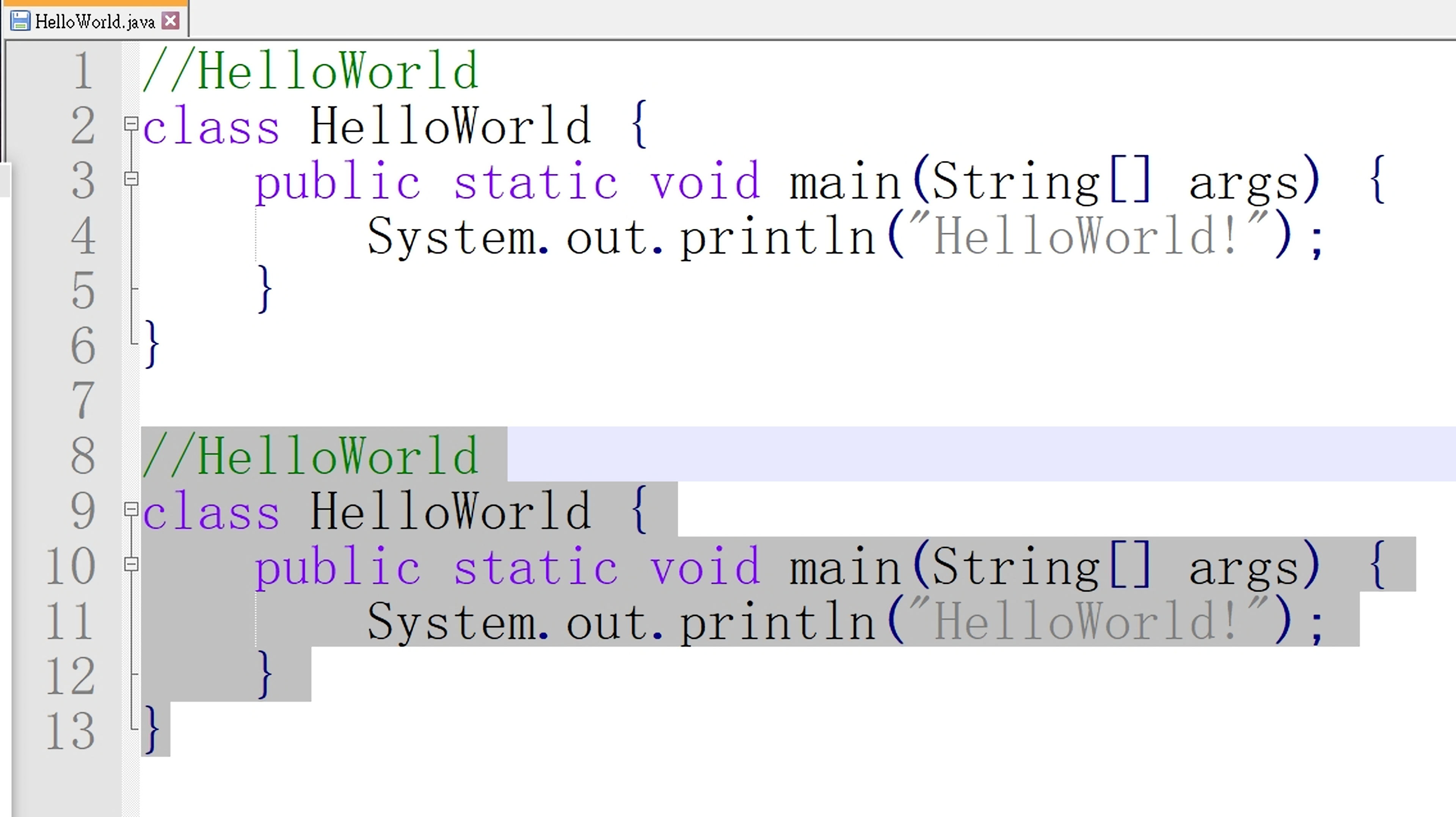Also these yellowish and blue strokes are part of the original image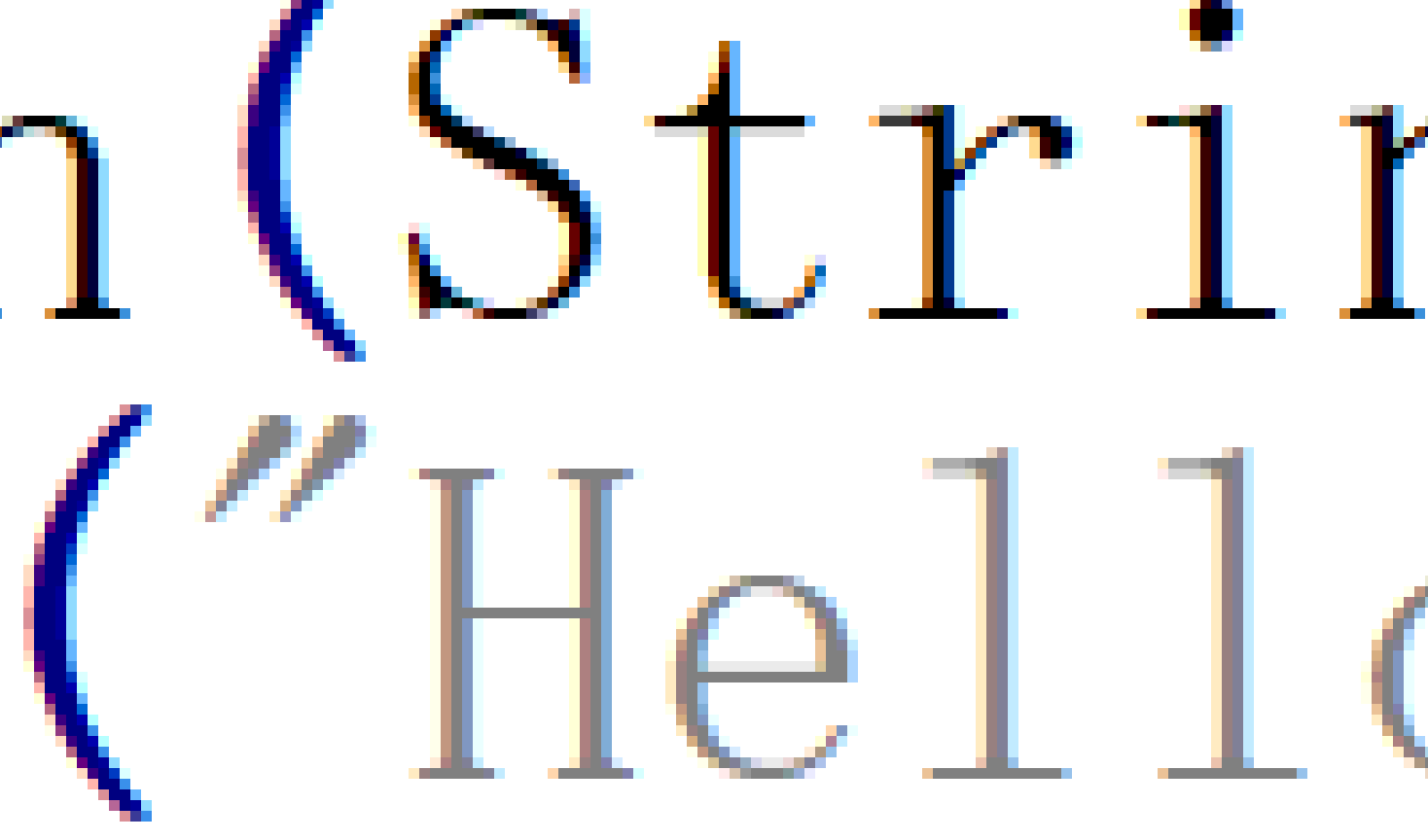Chroma bleeding sometimes happens with this shader, but FWIW it's mostly with a low bit-rate / quality images.

###VEWION commented Dec 23, 2020

 I didn't realize that those things are part of the original image LOL. Looks like KrigBilateral is trying to approach the original (also colors such as purple of the image above) and madvr's Bilateral sharp is trying to please human eyes IMO.

###hooke007 commented Feb 17, 2021 • edited

 It seems krig cannot handle well when --video-rotate=90/270 is set in mpv.conf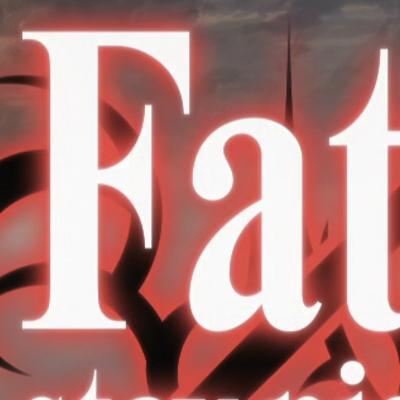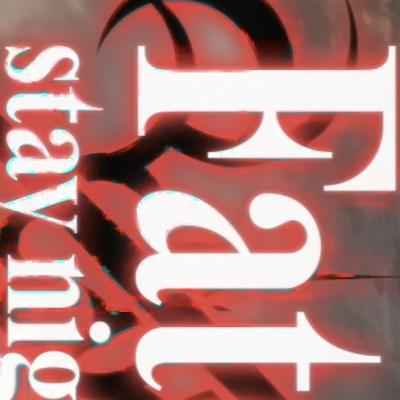###igv commented Feb 17, 2021

 Are you using hwdec? For me it happens only with hwdec and deband=no.

###hooke007 commented Feb 18, 2021

 Yes, using"--hwdec=auto-copy". Except bilinear/oversample/bicubic_fast, there is no other cscale filters can perfectly show the rotated videos.

###igv commented Feb 18, 2021

 It's all mpv bugs.
to join this conversation on GitHub. Already have an account? Sign in to comment